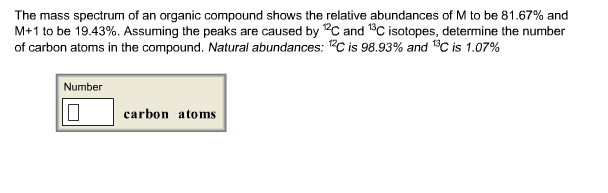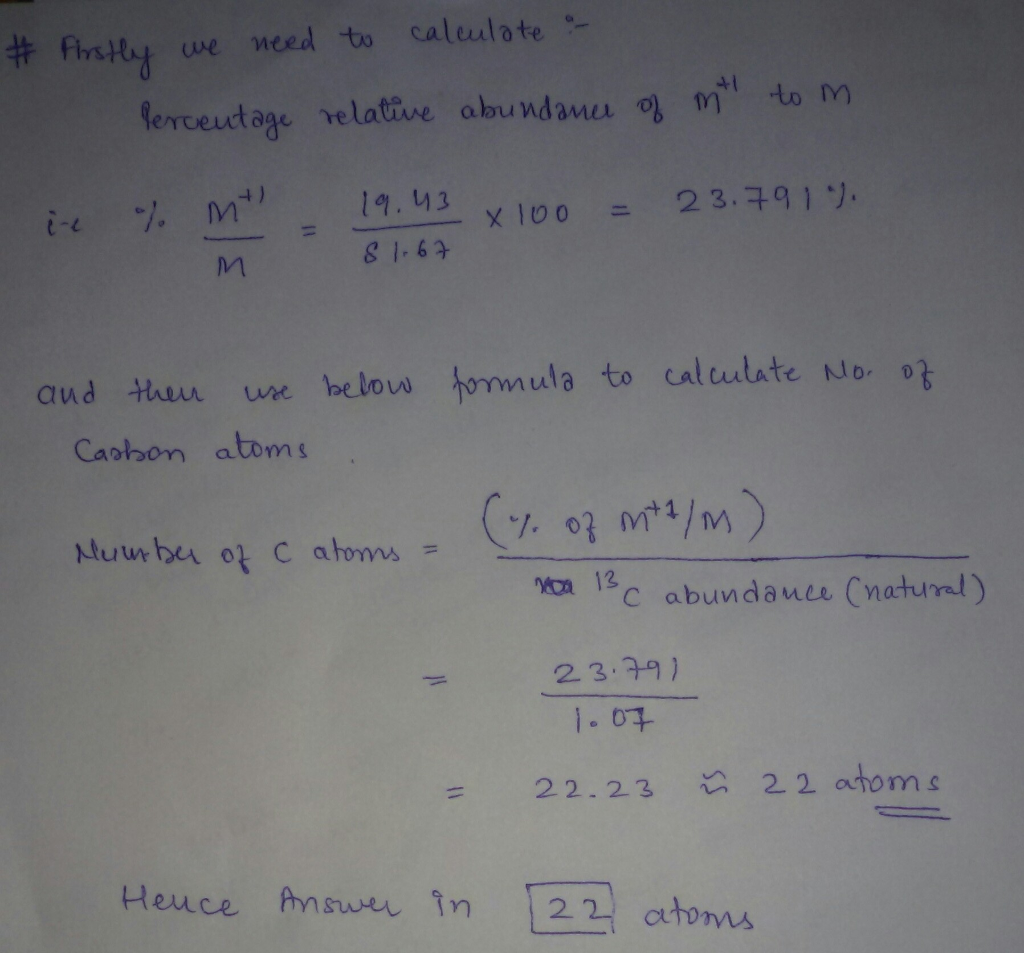# Question & Answer: The mass spectrum of an organic compound shows the relative abundances of M to be 81.67%…..The mass spectrum of an organic compound shows the relative abundances of M to be 81.67% and M + 1 to be 19.43%. Assuming the peaks are caused by ^12C and ^13C isotopes, determine the number of carbon atoms in the compound. Natural abundances: ^12 C is 98.93% and ^13C is 1.07%